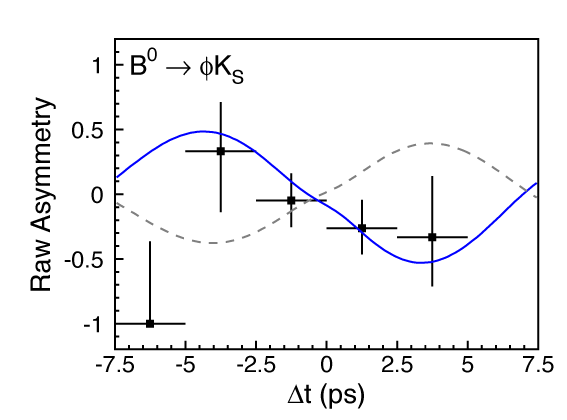## August 27, 2003

### Belle de Jour

There’s recent data out from BELLE (the B-meson factory at KEK) indicating new (beyond the Standard Model) CP-violating physics.Asymmetry data from BELLE, from which the value of $\sin(2\phi_1)$ is determined, plotted as a function of the decay time. The smooth dotted curve indicates what one would expect from the Standard Model, whereas the smooth solid curve is a fit to the observed data.

CP violation in the Standard Model is due to a single CP-violating phase in the Kobayashi-Maskawa matrix. CP violation has been known for a long time in the $K^0$ system. But, in the past couple of years, BELLE and BABAR (at SLAC) have been measuring CP violation in the $B^0_d$ system. As in the $K^0$ system the dominant CP-violating effect comes from $B^0\overline{B}^0$ mixing, and manifest itself in a time-dependence of the asymmetry $A(t) = \frac{\Gamma(\overline{B}^0\to f) - \Gamma(B^0\to f)}{\Gamma(\overline{B}^0\to f) + \Gamma(B^0\to f)}= \mp \sin(2\phi_1) \sin (\Delta{m} t)$ where the final state, $f$, is assumed to be a CP eigenstate with $\mathrm{CP} = \pm 1$ and $\phi_1$ is the relative phase between $V_{td}V_{tb}^*$ and $V_{cd}V_{cb}^*$.

Diagrams contributing to $B^0\to J/\Psi + K_S$

The asymmetry in $B^0 \to J/\Psi + K_S$ (never mind that $K_S$ is almost, but not quite a CP-eigenstate; that’s a negligible effect here) has been measured quite well by both BELLE and BABAR, and they agree $sin(2\phi_1)= +0.731\pm 0.056$ which is consistent with other measurements of CP violation and the assumption that it is all due to single CP-violating phase in the KM matrix.

But in $B^0\to \phi+ K_S$, BELLE finds a $3.5\sigma$ deviation from the Standard Model prediction. The decay is given, in the Standard Model, by a penguin diagram, of the form at right. To fit this to the BELLE data, would require $\sin(2\phi_1)= - 0.96\pm 0.50\pm 0.11$ which, as I said, is $3.5\sigma$ away from the above value. If this holds up, it’s clear indication there must be further sources of CP violation.

Penguin diagram contributing to $B^0\to \phi + K_S$

BABAR also has data on this process. Their result is more or less consistent with the Standard Model, $\sin(2\phi_1)=+0.45\pm0.43\pm 0.07$ That’s a $2.1\sigma$ discrepancy between the two experiments. If you average the two experiments (something I’m always suspicious of, but people do it all the time), you get $\sin(2\phi_1)= - 0.15\pm 0.33$, which is still $2.7\sigma$ from the Standard Model.

This could all still go away, but these penguin-diagram type processes are the ones most sensitive to new physics and there’s plenty of reason to expect new sources of CP violation in any extension (like supersymmetry) to the Standard Model. It would not be surprising if this were the first place where new physics were to reveal itself.

Or … this could all go away …

Posted by distler at August 27, 2003 12:22 AM

TrackBack URL for this Entry:   https://golem.ph.utexas.edu/cgi-bin/MT-3.0/dxy-tb.fcgi/209